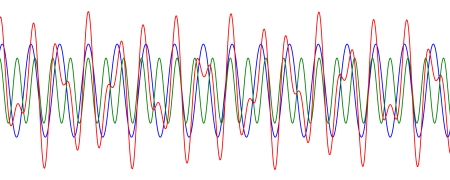# Linear ODEs with periodic solutions

Suppose we have a differential equation of the form

x” + b(t) x‘ + c(t) x = f(t)

where the functions b and c are periodic with the same period T.

When does the equation above have a solution with period T?

It turns out  the equation above will have a T-periodic solution for all T-periodic functions f if and only if the equation

x” + b(t) x‘ + c(t) x = 0

does not have a T-periodic solution. In other words, the non-homogeneous equation has a T-periodic solutions for all f if and only if the homogeneous equation does not .

This theorem may not sound right at first. You might think the homogeneous equation having a periodic solution would help if you were looking for a solution to the non-homogeneous equation. An example will show this isn’t the case.

## Example

Let’s look at the special case of b = 0, c = a positive constant, and f(t) = cos ωt. As shown here, the natural frequency of the equation is

ω0 = √c

and the homogeneous equation has general solution

x(t) = R cos(ω0t – φ)

for some amplitude R and phase φ.

Also, the non-homogeneous equation has a solution

cos(ωt) /(ω02 – ω2).

So does this contradict the theorem above? Here both the homogeneous and non-homogeneous equations have periodic solutions. However, they don’t have periodic solutions with the same period. The homogeneous solutions have period 2π/ω0, and the non-homogeneous solution has period 2π/ω.

The non-homogeneous solution above requires the driving frequency ω to be different from the natural frequency ω0 or else the denominator would be 0.

If the driving frequency does equal the natural frequency, the non-homogeneous equation does have a solution, but the solution is not periodic. The solutions blows up due to resonance. The non-homogeneous equation has a solution

(t/2ω) sin ωt.

and so the amplitudes of the oscillations grow as t grows. With each period, more energy is pumped into the system and the energy increases without bound.

## More general periods

The full set of solutions to a non-homogeneous equation is given by the general solution to the homogeneous equation plus any particular solution to the non-homogeneous equation.

If the driving frequency ω does not equal the natural frequency ω0 then in the example above the homogeneous and non-homogeneous solutions are both periodic, but with different periods. Is their sum periodic? Maybe.

The sum of two periodic functions may or may not be periodic. The image below is from this post where I give an example of two periodic functions, the green and blue lines, whose sum, the red line, is not periodic but rather quasiperiodic.The sum of two periodic functions is periodic if the ratio of their periods is a rational number. In the example above, the ratio is the golden ratio, an irrational number.

The general solutions to the non-homogeneous equation above are periodic if and only if the ratio of ω to ω0 is rational.

But if ω/ω0 is rational, do we have a contradiction to our theorem above? For example, suppose the natural frequency ω0 is 2π and the driving frequency ω is 4π. The the homogeneous solutions have period 1 and the particular solution to the non-homogeneous equation has period 1/2. But a function of period 1/2 also has period 1.

In our case, the equation

x” + 4π² x = 0

has solutions of period 1, and the equation

x” + 4π² x = cos 4πt

also has a solution with period 1.

To see why this isn’t a contradiction, we have to go back and read the theorem more carefully. It says the equation

x” + b(t) x‘ + c(t) x = f(t)

has a T-periodic solution for all T-periodic functions f if and only if the equation

x” + b(t) x‘ + c(t) x = 0

does not have a T-periodic solution.

In our case the homogeneous equation has solutions with period 1, and the non-homogeneous equation also has a solution with period 1. But this is just for one particular forcing function f. The theorem makes a claim for all functions f, and so we have not found a contradiction.

As long as there is some function f(t) with period 1 for which the non-homogeneous equation has no solution with period 1 then the theorem is correct. And in fact we’ve already given an example of such a function, namely cos 2πt. In that case the natural frequency and driving frequency are the same, and the solutions are not periodic due to resonance.

## Related posts

 See Topics in Ordinary Differential Equations by William Lakin and David Sanchez.

 A differential equation in the form above is called homogeneous if the forcing function on the right side is 0 and it is called non-homogeneous if the forcing function is not zero.

 If the driving frequency ω does not equal the natural frequency ω0 is the solution periodic even if it doesn’t have the same period